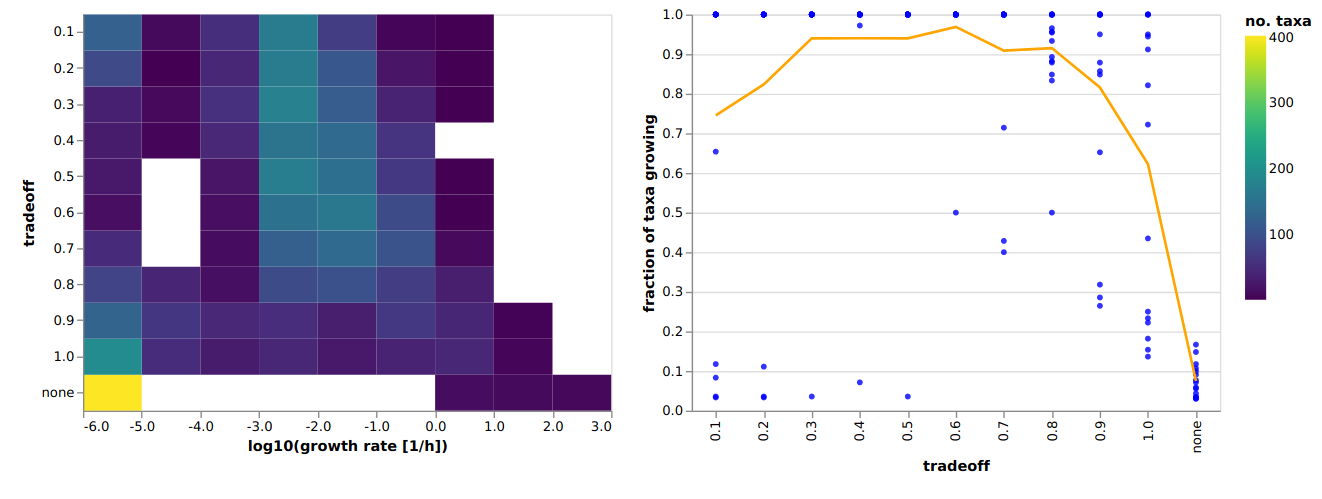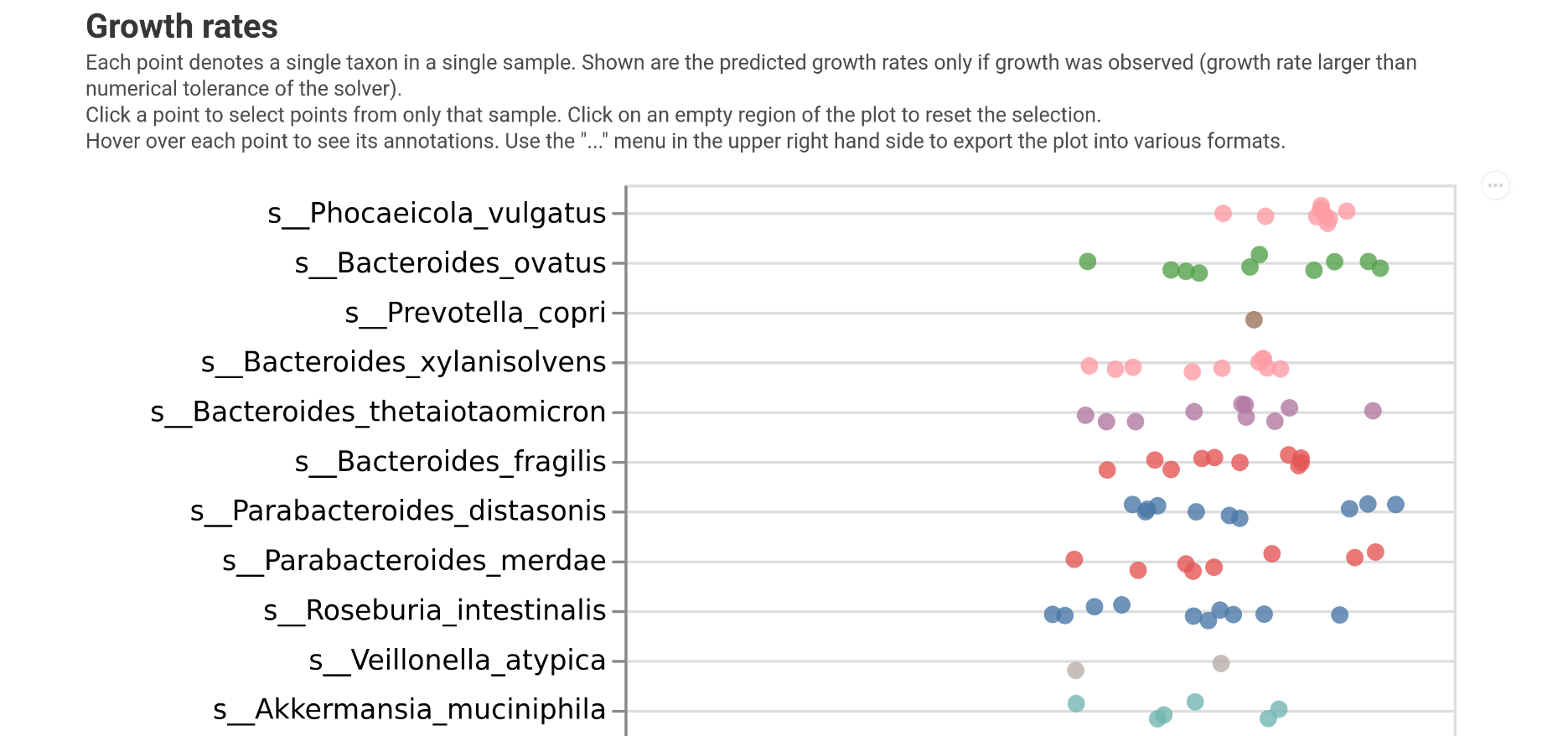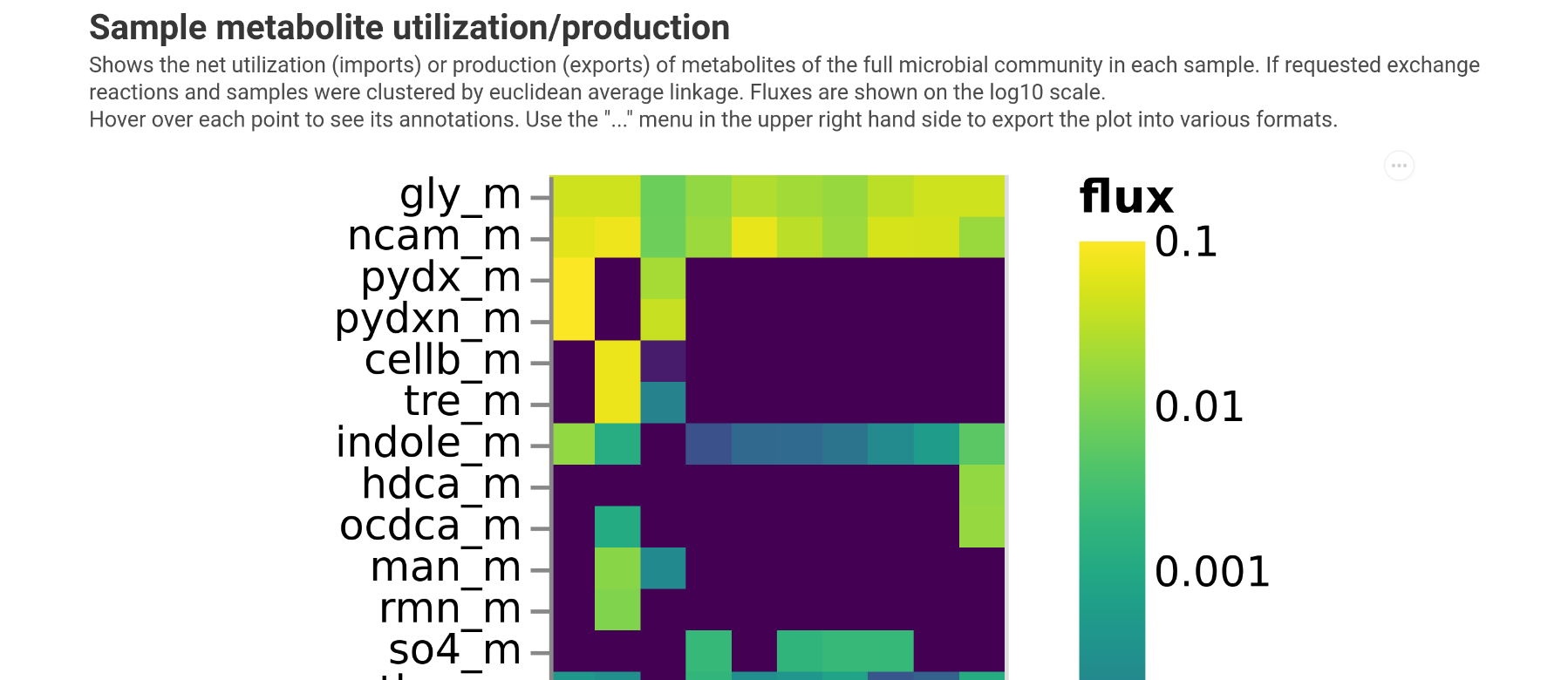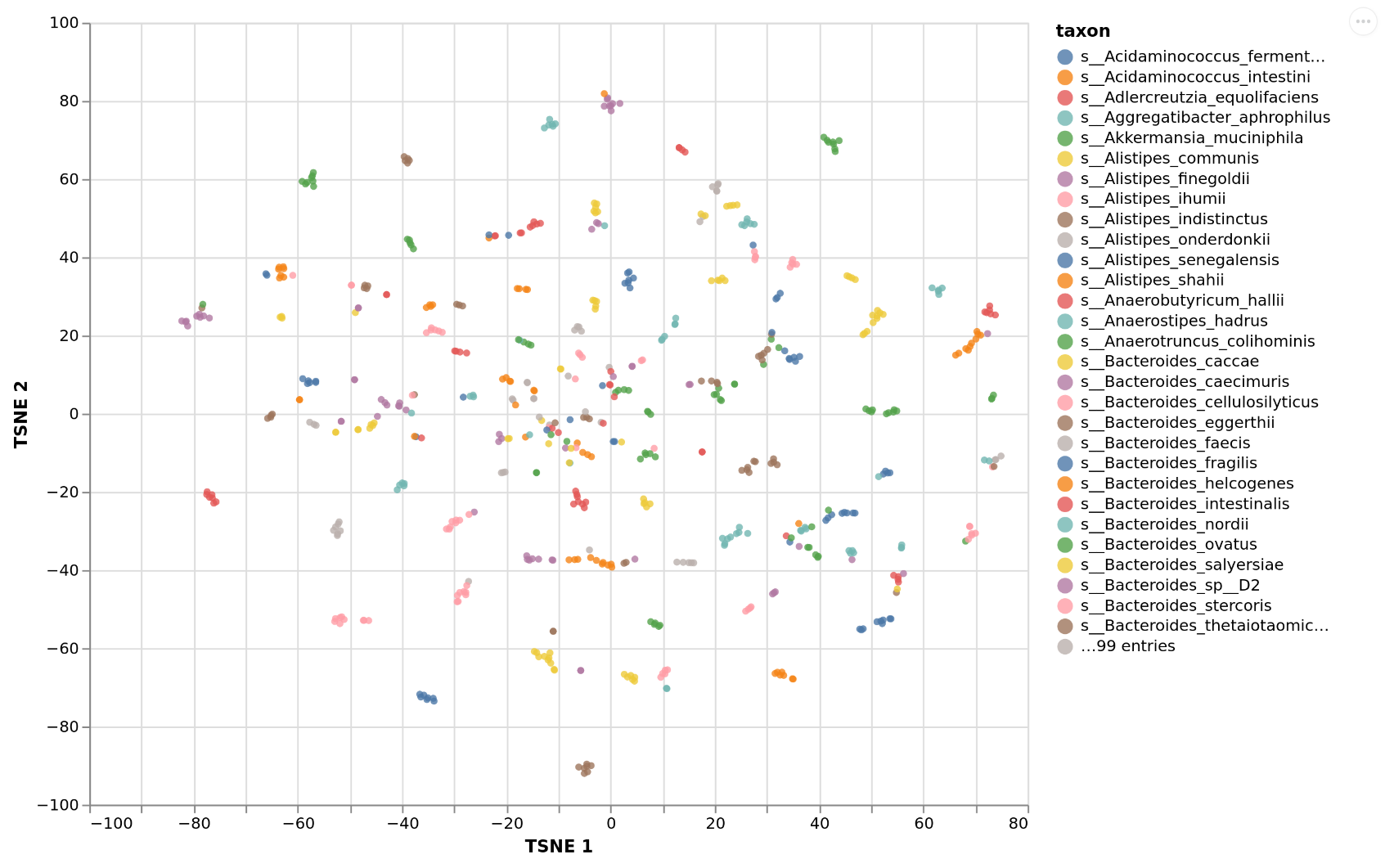# Visualizations¶

MICOM provides a a set of visualizations that can be used with the outputs from MICOM workflows. Those visualizations are the same as provided by the MICOM Qiime 2 plugin but are delivered as single HTML files that bundles interactive graphics and raw data.

To create some more interesting figures here we will use a realistic example data set which is the output of running the MICOM grow workflow on a data set of 8 healthy fecal samples and 8 fecal samples from individuals with colorectal cancer taken from https://doi.org/10.1158/1940-6207.CAPR-14-0129. To see the interactive evrsion of a visualization you can click on the provided previews. All visualization contain download buttons to download the raw data used to generate the plot.

:

import micom.data as mmd

crc_results = mmd.crc_results()


In the original MICOM publication we chose the tradeoff based on comparisons with in vivo replication rates derived from metagenome data. However, we observed that the highest correlation with replication rates is usually achieved at the largest tradeoff value that allows the majority of the taxa to grow. Thus, we can run cooperative tradeoff with varying tradeoff values and look for the characteristic elbow where the majority of the community can grow. This can be done by using the plot_tradeoff function.

:

from micom.viz import plot_tradeoff



The returned object is a Visualization object that contains the raw data in the data attribute.

:

pl

:

<micom.viz.core.Visualization at 0x7f17f0774490>

:

pl.data.keys()

:

dict_keys(['tradeoff'])


You could open the visualization in your browser with pl.view(). Alternatively you can just open the generated HTML file which would give you something like this:## Plotting growth rates¶

The first thing we may want to investigate are the growth rates predicted by MICOM. This can be done with the plot_growth function.

:

from micom.viz import plot_growth

pl = plot_growth(crc_results, filename="growth_rates.html")


Which will give you the following:## Plotting consumed metabolites¶

To get an overview which metabolites are consumed by the entire microbiota we can use the plot_exchanges_per_sample function.

:

from micom.viz import plot_exchanges_per_sample

pl = plot_exchanges_per_sample(crc_results, filename="consumption.html")


This will give you a heatmap showing all consumed components. Unless specified otherwise in the function arguments samples will be clustered so that samples with similar consumption profiles will be close.## Plotting growth niches¶

What is consumed globally may be interesting but we may want to know even more how the available growth niches are occupied by the taxa in the sample. This can be done with plot_exchanges_per_taxon which will embed the import fluxes for each taxon into two dimension using TSNE and plot the niche occupation map. Here taxa that overlap compete for similar sets of resources. The center of the map denotes the most competitive niche whereas the outskirts of the map denote more specialized consumption preferences.

:

from micom.viz import plot_exchanges_per_taxon

pl = plot_exchanges_per_taxon(crc_results, filename="niche.html")


This will give you the following:## Investigating the relationship with the phenotype¶

Finally we may want to which functions relate to an observed phenotype. This can be done with the plot_fit function which will:

1. calculate overall production fluxes (total metabolite amount produced by the microbiota)

2. log-transform and standardize production fluxes

3. train a LASSO regression (continuous response) or LASSO logistic regression (binary response)

4. present the coefficients and differences in production fluxes

:

from micom.viz import plot_fit
import pandas as pd

samples = pd.Series(crc_results.growth_rates.sample_id.unique())
status = pd.Series("healthy", index=samples)
status[status.index.str.startswith("Cancer")] = "colorectal cancer"
pl = plot_fit(crc_results, phenotype=status, variable_type="binary", filename="fit.html", min_coef=1e-3)


The output will look something like the following.So we see some higher sulfate production in cancer cases but also production of harmful components as TMAO. However, with the low sample numbers we don’t get a very good prediction based on fluxes.

[ ]: